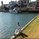10041 görüntülenme
10041
Small modification to stock UO code to highlight lows / highs. This helps to see the trend easily. The length to check for high/low is configurable (lengthSlope), I am yet to find the optimal setting but 30 looks nice so far.
```//
// @author LazyBear
// @credits This is derived from the stock UO code at TV
//
study(title="Ultimate Oscillator Bars [LazyBear]", shorttitle="UO_BARS [LazyBear]")
length7 = input(7, minval=1), length14 = input(14, minval=1), length28 = input(28, minval=1)
lengthSlope = input(1)
average(bp, tr_, length) => sum(bp, length) / sum(tr_, length)
high_ = max(high, close)
low_ = min(low, close)
bp = close - low_
tr_ = high_ - low_
avg7 = average(bp, tr_, length7)
avg14 = average(bp, tr_, length14)
avg28 = average(bp, tr_, length28)
out = 100 * (4*avg7 + 2*avg14 + avg28)/7
plot(out, color=red, title="UO")
bgcolor(falling(out, lengthSlope) ? red : (rising(out, lengthSlope) ? green : blue), transp=50)```Thanks got it.. I am not a coder but looking at all the codes some day I might become a pseudocoder...
Cevap Gönderhow does this work. I mean how to use this Indicator.
Cevap Gönder